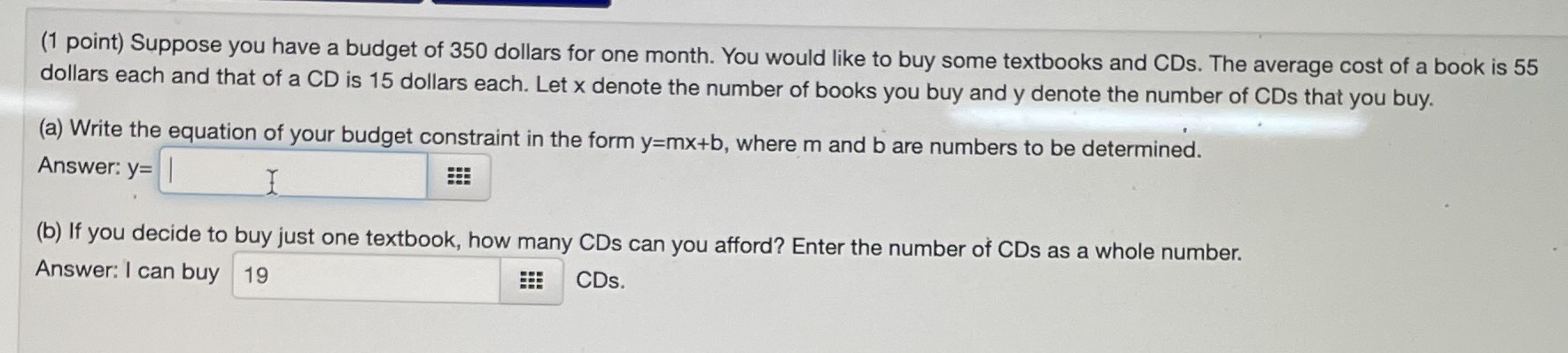### ¿Todavía tienes preguntas de matemáticas?

Pregunte a nuestros tutores expertos
Algebra
Pregunta(1 point) Suppose you have a budget of $$350$$ dollars for one month. You would like to buy some textbooks and CDs. The average cost of a book is $$55$$ dollars each and that of a CD is $$15$$ dollars each. Let x denote the number of books you buy and y denote the number of CDs that you buy.

(a) Write the equation of your budget constraint in the form $$y = m x + b$$ , where $$m$$ and b are numbers to be determined. Answer: y=________?

(b) If you decide to buy just one textbook, how many CDs can you afford? Enter the number of CDs as a whole number. Answer: I can buy ________CDs.

a) $$y= -\frac{11}{3}x+ \frac{70}{3}$$